﻿ Reverse Rules

# Reverse Rules and Data-Entry Simulation

A reverse rule is a rule which specific syntax enables the capability to trigger multiple data saving on several items.

## Defining a Reverse Rule - Difference with Simple Rule

The Reverse Rule is recognized when the formula is preceded by the "pipe" symbol ( | ). Reverse Rules can coexist with simply Rule in the same Item Rule definition.

Let's consider the following sample :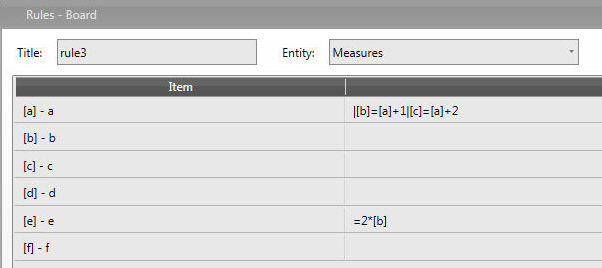As we input any figure for item [a] items [b],[c] and [e] are calculated and saved like a+1 and a+2 respectively.

This action is analogue to the following simple Rule  but data for items [b],[c] and [e] are calculated and visualized but not saved.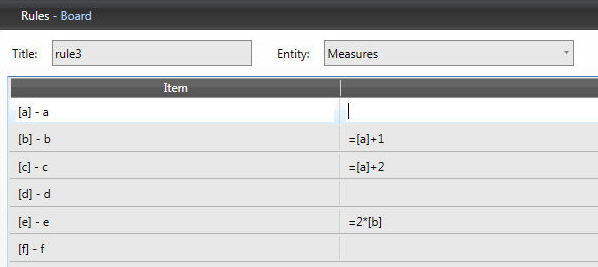Note

We can have unlimited number of reverse calculations in the same item row

We can use reverse rules in combination with simple rules; simple rule must precede Reverse Rules.

### Simple Rule prevails on Reverse Rule

Let's discuss the following sample :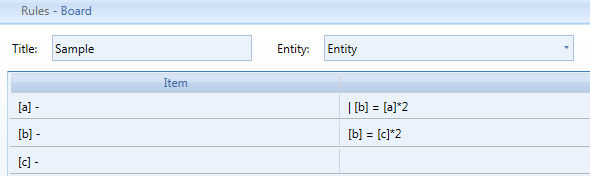The Saved Data in item [b] is equal to the double of [a] Data

The Visualized Data in  in item [b] is equal to the double of [c] Data

Attention ! There could be a misalignment between Saved Data and Visualized Data.

### Reverse Rule Order affects the Data Calculation

The Reverse Rule are executed from left to right. These two Rules provides different results :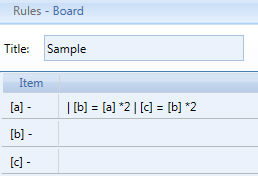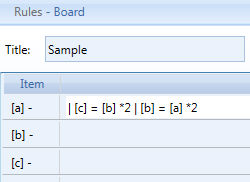(rule 1)                                                                            (rule 2)

Rule 1 : If [a] = 10, then [b] is 20(the double of [a]) and then [c] is 40 (the double of [b])

Rule 2 : If [a] = 10, then [c] is 0([b] is still zero !) and then [b] is 20 (the double of [a])

Note

The result of the Rule calculation is visible after you click on the Save Data Icon unless the Screen property Data-Entry Simulation is activated. Refreshing the Screen resets the Data